The fruit salad is prepared from oranges and bananas. One kilogram of oranges costs 30 CZK and one kilogram of bananas costs 18 CZK. How many kilograms of bananas need to be added to half a kilogram of oranges to produce a fruit salad worth CZK 21 per 1 kg?

Result

x =  1.5 kg

#### Solution:

18x + 30*0.5 = 21*(x+0.5)

3x = 4.5

x = 32 = 1.5

Calculated by our simple equation calculator.

Our examples were largely sent or created by pupils and students themselves. Therefore, we would be pleased if you could send us any errors you found, spelling mistakes, or rephasing the example. Thank you!

Leave us a comment of this math problem and its solution (i.e. if it is still somewhat unclear...):Be the first to comment!#### Following knowledge from mathematics are needed to solve this word math problem:

Do you have a linear equation or system of equations and looking for its solution? Or do you have quadratic equation?

## Next similar math problems:

1. Tea mixture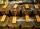Of the two sort of tea at a price of 180 CZK/kg and 240 CZK/kg we make a mixture 12 kg that should be prepared at a price of 200 CZK / kg. How many kilos of each sort of tea will we need to be mixed?
2. Acid evaporationHow many kilograms of water do we have to evaporate from 100 kg of 32% acid to make it 80% concentration?
3. Four friendsFour friends shared the money. Vasek got 1/4 of the total amount. Tonda received 1/3 of the rest of the money, Joe got a half from the second residue and Jirka left 80. How much money get together?
4. Distilled waterSea water contains 5% salt. How many distilled water should be pour to 40 kg of sea water so that the salt content is 2%. How many kilograms of 2% of sea water we get?
5. MixingIf we mix 5 kg of goods of one kind and 3 kg second one, resulting mixture cost 16.50 EUR/kg. If these quantities are mixed in reverse - first three kilograms and 5 kilograms second cost of mixture is 18.50 EUR/kg. What is the price of one kg of goods of.
6. CandiesThe price for 1 kg of more expensive candies is 125 CZK. The price for 1 kg of cheaper candy is 100 CZK. We mix two different mixtures of candy. A) The first mixture contains 2 kg more expensive and 0.5 kg cheaper candy. Calculate the price for 1 kg of t
7. CandyThe price for 1kg of more expensive candy is 125 CZK. The price for 1kg of cheaper candy is 100 CZK. We make two different blends of candy. And now. The second blend contains 2kg of more expensive candy and several kg of cheaper candy. The price per 1 kg o
8. Acid solutionBy adding 250 grams of a 96% solution of sulfuric acid to its 3% solution its initial concentration was changed to 25%. How many grams of 3% of the acid were used for dilution?
9. BanknotesEva deposit 7800 USD in 50 banknotes in the bank. They had value 100 USD and 200 USD. How many were they?
10. Three granaries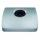Three granaries hold 7,800 metric cent (q) of grain. In the first, 70 tonnes of grain was more than the second and in the third it was 120 tonnes less than in the second. How many grain were in each granary?
11. Bakery and flour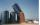The bakery tray for flour was filled to 3/4 volume. After removing 875 kg of flour, it was filled to only 2/5 of the volume. How many tons of flour is in the full tray?
12. Fifth of the numberThe fifth of the number is by 24 less than that number. What is the number?
13. SugarsIn what ratio must two sorts of sugar, costing #390 and #315 per kg respectively, be mixed in order to produce a mixture worth #369 per kg?
14. Copper sulphate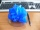How much g of water do we have to add to 240 g of an 84% CuSO4 solution to produce a 60% solution? (Express the mass of crystalline CuSO4 in the original solution and in the resulting solution and compare them. )
15. Water wessel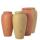The vessel containing water has a weight 19 kg. After pouring half the water its weigth 11 kg. What is the weight of the empty vessel?
16. On the 4On the way to playing disc golf with his two boys, Mr. Smith purchases 3 muffins and 2 bottles of water, totaling \$9.75. The following week he only has Asher with him, so he purchases 2 muffins and 1 bottle of water totalling \$6.00. What is the cost of on
17. 12 apples12 apples and 2 loaves of bread cost 5.76 and 6 apples and 3 loaves of bread cost 7.68. How much is a loaf of bread?